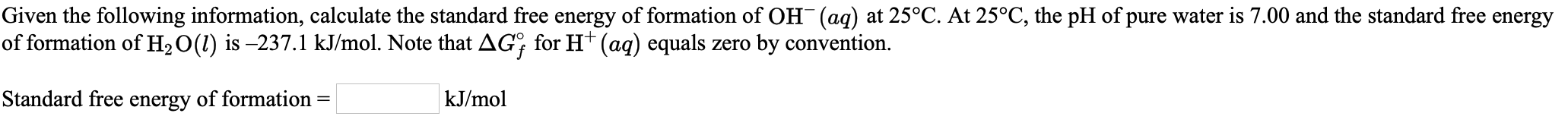# Given the following information, calculate the standard free energy of formation of OH-(aq) at 25°C. At 25°C, the pH of pure water is 7.00 and the standard free energy formation of H2O (l) is -237.1 kJ/mol. Note that deltaGf for H+ equals zero by convention